# Potential Energy with Examples

Article Index
Potential Energy
Potential Energy Continue
All Pages

POTENTIAL ENERGY

Objects have energy because of their positions relative to other objects. We call this energy as potential energy. For example, apples on the tree, or compressed spring or a stone thrown from any height with respect to ground are examples of potential energy. In all these examples there is a potential to do work. If we release the spring it does work or if we drop the apples they do work. To move the objects or elevate them with respect to the ground we do work. The energy of the objects due to their positions with respect to the ground is called gravitational potential energy.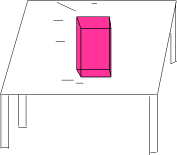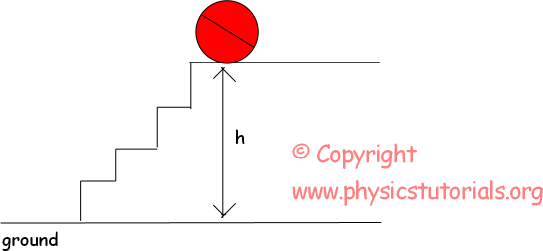The pictures given above are the examples of gravitational potential energy. They both have a height from the ground and because of their positions they have energy or potential to do work. Look at the given examples below. They are a little bit different that of given above.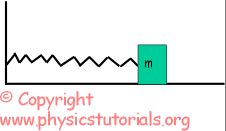In the first picture, system including a spring and a box is at rest. However, in second picture the box compresses the spring and loads it with potential energy. If we release the box spring does work and pushes the box back. These two examples of gravitational and spring potential energy are calculated differently. Let me begin with the calculation of gravitational potential energy. We will look at which factors effects the magnitude of potential energy or which does not effect. Work done against to the earth to elevate the objects is multiplication of its weight and distance which is height. Thus, as we said before energy is the potential of doing work.

Then, gravitational potential energy becomes;

PE=mg.h

Now, look at the given picture and try to calculate the potential energies of the given ball in three situations.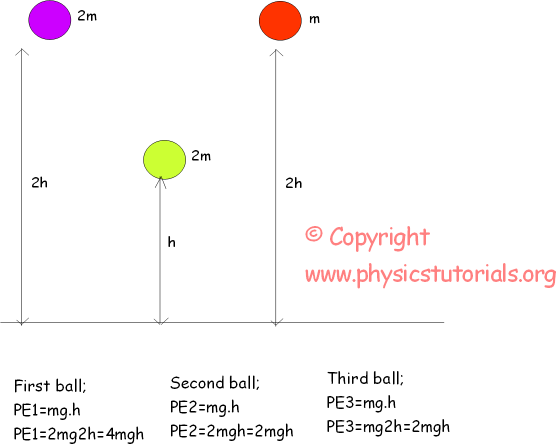We see that gravitational potential energy depends on the weight and height of the object. Now let’s solve some more examples related to this topic before passing to the kinetic energy.

Example In the pictures given below, if the potential energy of the ball in the first picture is P find the potential energy of the ball in second situation in terms of P.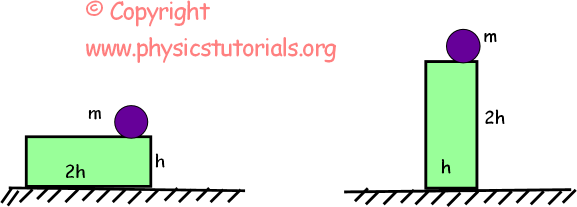Situation 1 Situation 2

P=m.g.h=mgh P’=m.g.2h=2mgh=2P

Related